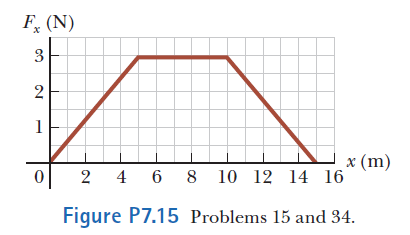# Problem: A 4.00-kg particle is subject to a net force that varies with position as shown in the figure. The particle starts from rest at x 5 0. What is its speed at (a) x = 5.00 m, (b) x = 10.0 m, and (c) x = 15.0 m?

83% (67 ratings)
###### Problem Details

A 4.00-kg particle is subject to a net force that varies with position as shown in the figure. The particle starts from rest at x 5 0. What is its speed at (a) x = 5.00 m, (b) x = 10.0 m, and (c) x = 15.0 m?What scientific concept do you need to know in order to solve this problem?

Our tutors have indicated that to solve this problem you will need to apply the Work By Variable Forces (Springs) concept. You can view video lessons to learn Work By Variable Forces (Springs). Or if you need more Work By Variable Forces (Springs) practice, you can also practice Work By Variable Forces (Springs) practice problems.

How long does this problem take to solve?

Our expert Physics tutor, Julia took 5 minutes and 2 seconds to solve this problem. You can follow their steps in the video explanation above.

What professor is this problem relevant for?

Based on our data, we think this problem is relevant for Professor Efthimiou's class at UCF.

What textbook is this problem found in?

Our data indicates that this problem or a close variation was asked in Physics for Scientists and Engineers - Serway Calc 9th Edition. You can also practice Physics for Scientists and Engineers - Serway Calc 9th Edition practice problems.